You are not logged in. Please login at www.codechef.com to post your questions!

×

# FUNAGP - Editorial

Author: Devendra Agarwal
Tester: Sergey Kulik
Editorialist: Lalit Kundu

HARD

# PREREQUISITES:

Binary Indexed Trees/Segment Trees
Mathematics and Number Theory

# PROBLEM:

Given fixed parameters R, p1, p2 (p1,p2 are primes) and array A, perform three operations:

* 0 S D X Y: Add an AGP with the start term of S, the common difference of D, common ratio of R from the X-th to the to Y-th element of A.

* 1 X g: A[X] = (A[X])g modulo p2.

* 2 X Y: Output (A[X] + ...... + A[Y]) modulo p1.

Array A can have upto 105 elements and upto 105 queries.

# EXPLANATION:

We can see AGP as an infinite AGP. To add an AGP from [X,Y] is equivalent to adding 2 inﬁnite AGP’s which are:
(a) Start Term = S, Common Difference = D and Starting from X.
(b) Start Term = −(S + (Y − X + 1) * D) * RY-X+1, Common Difference = −D * RY-X+1 and starting from Y+1.

Now the solver can use any data structure such as BIT/segment tree. But since BIT are easy to implement and efficient, I will discuss the approach using BIT.

Supose that using BIT, we somehow solve queries 0 and 2, how can we solve query 1 efficiently. Here we keep two BIT, one is modulo p1 (say BIT1) and other modulo p2 (say BIT2). For query 1 ie. 1 X g

// read respective values at X

// update to make both indexes 0
update(BIT1,X,-var1)
update(BIT2,X,-var2)

// update to add new values
update(BIT1,X,var2^g mod p2)
update(BIT2,X,var2^g mod p2)


Now, we have to answer queries 0 and 2. Let us analyse the situation after just one addition of query 0 (which is added at X, as we have broken 1 AGP into 2 infinite AGp’s).

Let us find sum of all elements from 1 to J.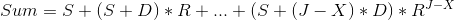Multiplying with R, we get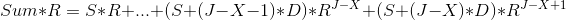Subtracting above 2 equations, we get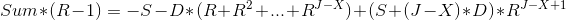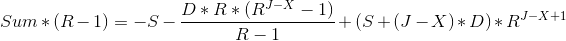Simplifying, we get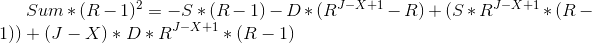Let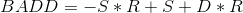Let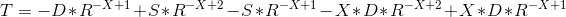Let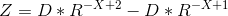# Note:

(a) BADD, T and Z are independent of query point J.
(b)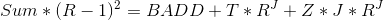By using Fact (a), we can store BADD, T and Z in the BIT (as it is independent of query point) in the update query 0 and use fact (b) for query of type 2.

Solution is not yet complete as we need to augment our data structure with yet another field ADD, which keeps the constant addition of the value in the index. This is used intially and for query 1. So, the output of query 2 changes from (b) to:

(c)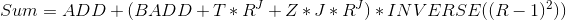Wait, the solution is not yet over! What if (R-1)2 is not coprime to prime numbers, then we won't be able to find INVERSE of it and hence our solution will fail. In this case, the problem transforms to AP as R mod prime = 1 mod prime.

Solve for AP in the similar idea using data structures.

Complexity of solution is O( N * log(N) ) intially, plus O ( log(N) ) for each query. So overall complexity is O( (N+Q)* log(N)).

See setter's solution for implementation details using the above idea.

# ALTERNATE SOLUTION:

(as told by @triveni)
There was no need of computing any kind of inverse modulo or such things at all to solve this problem, using the method that I followed. Even if p1 and p2 are not primes, this problem can be solved very easily using the same approach. I used Segment Tree data Structure. In my opinion, the hardest part to solve this problem is to find the sum of the AGP in efficiently. It is said in the editorial, we need to compute Modulo((R-1)**2).

But actually we can do something better here.

sum(i) = S + (S+D)*R + (S+2D)*R^2 + (S+3D)*R^3 +....+ (S+(i-1)*D)*R^(i-1)

=>sum(i) = (S + SR + SR^2 + SR^3 + SR^4 +....) + (0 + DR + 2DR^2 + 3DR^3 + 4DR^4 +....)

=>sum(i) = S(1 + R + R^2 + R^3 + R^4 +...) + D(0 + R + 2R^2 + 3R^3 + 4R^4 +...)
=>sum(i) = S * X(i) + D * Y(i)

Now, what one can do is pretty simple. Just pre-compute the value of X(i) and Y(i) for all 1<=i<=N.
Hence, it becomes pretty easy. Here is my solution using segment tree approach http://www.codechef.com/viewsolution/3844832.

This method doesn't assume anything about primeness of p1 and p2.

# AUTHOR'S AND TESTER'S SOLUTIONS:

This question is marked "community wiki".

asked 12 May '14, 15:143.0k93164187
accept rate: 12%

^I'm in exactly the same situation. Maybe it has to do with this part "What if (R-1)2 is not coprime to prime numbers". Though my solution http://www.codechef.com/viewsolution/3900035 (solved using sqrt-decomposition) gives a WA regardless. Surprisingly no AC solution uses square root decomposition.

Also some cheating cases: http://www.codechef.com/viewsolution/3875556 http://www.codechef.com/viewsolution/3878413

(12 May '14, 17:39)

Report cheating cases to admin via mail.

(12 May '14, 18:07)

Can you explain in detail how to solve when (R-1)^2 is not comprime to prime nos? How does the problem transform to an AP?

(13 May '14, 01:46) 5★

(R-1)^2 not coprime to a prime p means that gcd(p,(R-1)^2) > 1. However, since p is prime, that means gcd can only be p, so p|(R-1)^2. This is equivalent to saying R = 1 mod p, which means that S+(S+D)R + (S+2D)R^2 + ... + (S+nD)R^n = S+(S+D)+(S+2D)+...+(S+nD) mod p

(13 May '14, 02:18) 7★

got it, thanks!

(13 May '14, 14:42) 5★

@all @darkshadows Please explain the complexity of query type 0 . By my understanding, if query of type 0 starts from 1 and end at N then updating the bit will be of O(N * logN ) [No of elements to update * complexity of one update], so if there are M queries of type 0 then compelity can be M * N * logN which will exceed the time limit.

(27 May '14, 13:28)

@devuy11 can you please explain this ?

(28 May '14, 09:40)
showing 5 of 7 show all

 15 There was no need of computing any kind of inverse modulo or such things at all to solve this problem, using the method that I followed. Even if p1 and p2 are not primes, this problem can be solved very easily using the same approach. I used Segment Tree data Structure. In my opinion, the hardest part to solve this problem is to find the sum of the AGP in efficiently. It is said in the editorial, we need to compute Modulo((R-1)**2). But actually we can do something better here.       sum(i) = S + (S+D)R + (S+2D)R^2 + (S+3D)R^3 +....+ (S+(i-1)D)R^(i-1) =>sum(i) = (S + SR + SR^2 + SR^3 + SR^4 +....) + (0 + DR + 2DR^2 + 3DR^3 + 4DR^4 +....) =>sum(i) = S(1 + R + R^2 + R^3 + R^4 +...) + D(0 + R + 2R^2 + 3R^3 + 4R^4 +...) =>sum(i) = S * X(i) + D * Y(i) Now, what one can do is pretty simple. Just pre-compute the value of X(i) and Y(i) for all 1<=i<=N. Hence, it becomes pretty easy. Here is my solution using segment tree approach http://www.codechef.com/viewsolution/3844832. This method doesn't assume anything about primeness of p1 and p2. answered 13 May '14, 02:275★triveni 1.0k●1●4●14 accept rate: 12% very good solution that you have provided i also stucked in the same part but the way you solved it is amazing .. (13 May '14, 10:51) kodoos4★ great solution (13 May '14, 14:39) karan1735★ 2 I have also added this solution to editorial, thanks for the approach! (13 May '14, 15:46)
 0 May i know, for which testcase, it failed http://www.codechef.com/viewsolution/3872050 answered 12 May '14, 19:361●1●1 accept rate: 0% Hi miiitd6979672,  I think you have implemented the brute force solution to the problem which is not expected to pass because in worst case, total number of iterations will shoot up to (10^5) * (10^5).  (13 May '14, 20:07)
 0 If (R-1)^2 is coprime with P we still need to get R^(-X+1) but R might not be coprime with P in which case R^(-X+1) does not exist. I didn't get how this solution deals with it. For instance, R = 9 and P = 3, if we got a query with X = 9, won't we need 9^-8 mod 3? answered 13 May '14, 07:091●1 accept rate: 0% 2 As p1 and p2 are primes , there are 2 cases(considering only p1) Case 1: p1 is a prime , p1 divides R , in that case the series becomes S Case 2: p1 is a prime , R is coprime to p1 , so you can find inverse modulo. (13 May '14, 08:21)
 0 @all As we all know there are many ways to solve this problem .we can use any of the two available data structures BIT/segment tree. BIT solution is described by @darkshadows and an easy approach is descibed by triveni to handle complex part in this question but i dont know why i am only able to get AC using triveni's approach and if i am using modulo inverse approach i am continuously getting WA. can any one check this solution and tell me where is the problem http://www.codechef.com/viewsolution/3902074 +1 to the problem setter +1 to the darkshadows for providing approach using BIT +1 to the triveni answered 13 May '14, 13:344★kodoos 1●1 accept rate: 0% Hi kodos, You are getting Wrong Answer for the test case  1 3 2 2 17 2 0 0 0 0 1 1 1 3 2 1 3 Answer should be 0 and you are getting 4 (13 May '14, 17:03) @all @darkshadows Please explain the complexity of query type 0 . By my understanding, if query of type 0 starts from 1 and end at N then updating the bit will be of O(N * logN ) [No of elements to update * complexity of one update], so if there are M queries of type 0 then compelity can be M * N * logN which will exceed the time limit. (27 May '14, 13:28)
 toggle preview community wiki:
Preview

### Follow this question

By Email:

Once you sign in you will be able to subscribe for any updates here

Markdown Basics

• *italic* or _italic_
• **bold** or __bold__
• image?![alt text](/path/img.jpg "title")
• numbered list: 1. Foo 2. Bar
• to add a line break simply add two spaces to where you would like the new line to be.
• basic HTML tags are also supported
• mathemetical formulas in Latex between \$ symbol

Question tags:

×15,852
×1,768
×1,359
×371
×20
×2

question asked: 12 May '14, 15:14

question was seen: 6,091 times

last updated: 28 May '14, 09:40